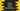# 3 ways to create a list of alternate elements in Python## Python program to create a list of alternate elements:

There are many ways in python that can be used to create a separate list by picking the alternate elements. We don’t need to iterate through the list using a loop or anything like that.

We can use slicing or we can use list comprehension. In this post,

## Method 1: By using list slicing:

Let’s try to solve this by using slicing. list slice function is defined as below:

``list[start: end: step]``

Here,

• start is the index position to start the slicing. By default it is 0.
• end is the index position to end the slicing. If we don’t provide this value, it takes the length of the list.
• step is the number of elements to skip while slicing.

This method returns a new list with the new elements.

Let’s use slicing to pick the alternate elements from a list:

### Python program:

``````given_list = [1, 2, 3, 4, 5, 6, 7, 8, 9, 10]

new_list = given_list[::2]

print(new_list)``````

In this program, we are not passing the start and the end index position, but we are passing the step as 2. If you run this program, it will print the below output:

``[1, 3, 5, 7, 9]``

If you want to start from the second element, you can provide the start index for that:

``````given_list = [1, 2, 3, 4, 5, 6, 7, 8, 9, 10]

new_list = given_list[1::2]

print(new_list)``````

It will print:

``[2, 4, 6, 8, 10]``

## Method 2: By using list comprehension:

List comprehension is another way to quickly pick the alternate numbers from a list. It is a shorthand of using a loop to iterate and pick the numbers.

Let’s write down the program using list comprehension:

``````given_list = [1, 2, 3, 4, 5, 6, 7, 8, 9, 10]

new_list = [given_list[i] for i in range(len(given_list)) if i % 2 != 0]

print(new_list)``````

This program will pick only the odd index numbers from this list. If you run this program, it will print the below output:

``[2, 4, 6, 8, 10]``

Similarly, we can also change it to pick only the even index numbers:

``````given_list = [1, 2, 3, 4, 5, 6, 7, 8, 9, 10]

new_list = [given_list[i] for i in range(len(given_list)) if i % 2 == 0]

print(new_list)``````

It will print:

``[1, 3, 5, 7, 9]``

## Method 3: By using filter:

filter method takes one iterable and using one function, it creates one iterator. Below is the syntax of this method:

``filter(function, iterable)``

The function returns one boolean value. For those elements for which this function returns true are picked. We can pass one lambda as the first parameter. Below is the complete program:

``````given_list = [1, 2, 3, 4, 5, 6, 7, 8, 9, 10]

new_list = list(filter(lambda x: x % 2 == 0, given_list))

print(new_list)``````

It will pick only the even numbers from the list. If you run this program, it will print the below output:

``[2, 4, 6, 8, 10]``

This method works if you want to check the numbers and pick them. If you want to check the index values, you can use any one of the first and second method.